Subtraction Board Games (Numbers to 100) (SB7271) - SparkleBox

Find Resources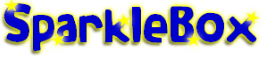Subtraction Board Games (Numbers to 100)

# Subtraction Board Games (Numbers to 100)

Description

Simple board games where children move around the board and answer the subtraction number sentences.

Simple board games where children move around the board and answer the subtraction number sentences.

Preview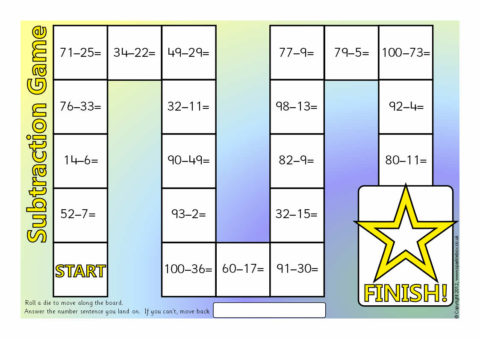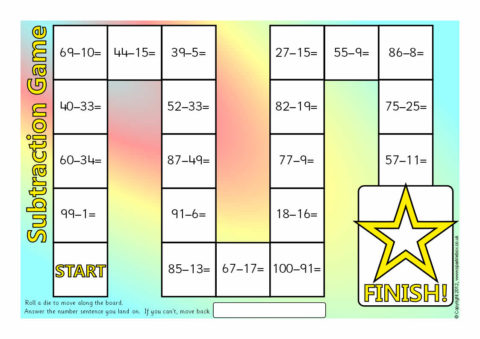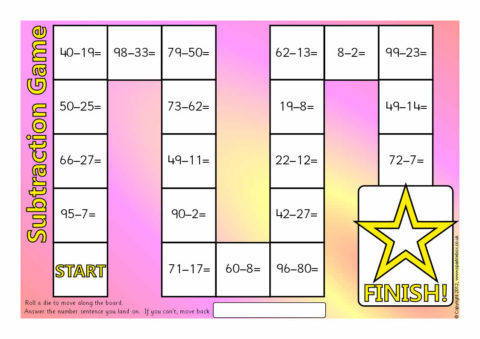# Related Items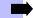Subtraction Resources